## Which explicit formula is equivalent to a1 = 1, an= 4an-1? A. an = 1(4)^n-1 B. an = 4(4)^n-1 C. an = 4(1)^n–1

Question

Which explicit formula is equivalent to a1 = 1, an=
4an-1?
A. an = 1(4)^n-1
B. an = 4(4)^n-1
C. an = 4(1)^n–1
D. an = 1 + (n – 1)4

in progress 0
2 weeks 2021-09-05T14:47:13+00:00 1 Answers 0 views 0

A. an = 1(4)^n-1

Step-by-step explanation:

Geometric sequence:

In a geometric sequence, the quotient between consecutive terms is always the same, called common ratio, and the sequence is given by: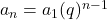In which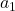is the first term and q is the common ratio.

We are given the following sequence: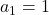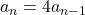So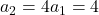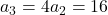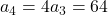That is, the quotient between consecutive terms is 4, so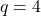.

The first term is already given,. So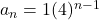And thus, the correct answer is given by option A.